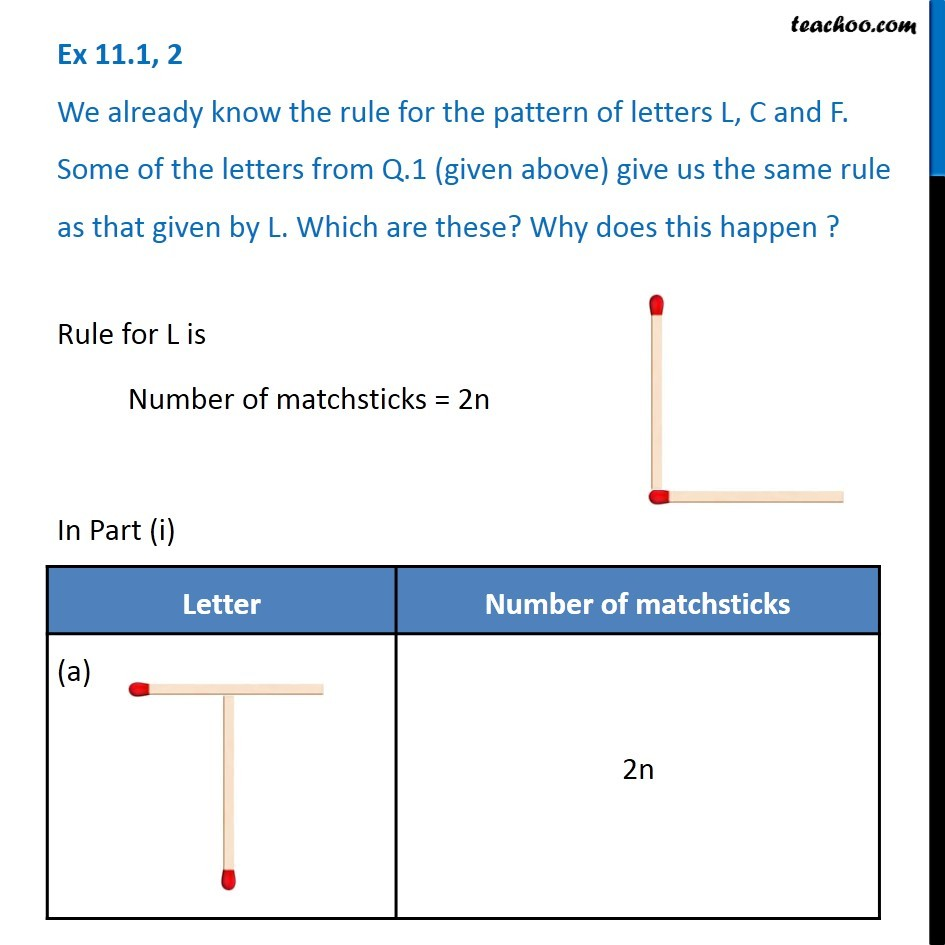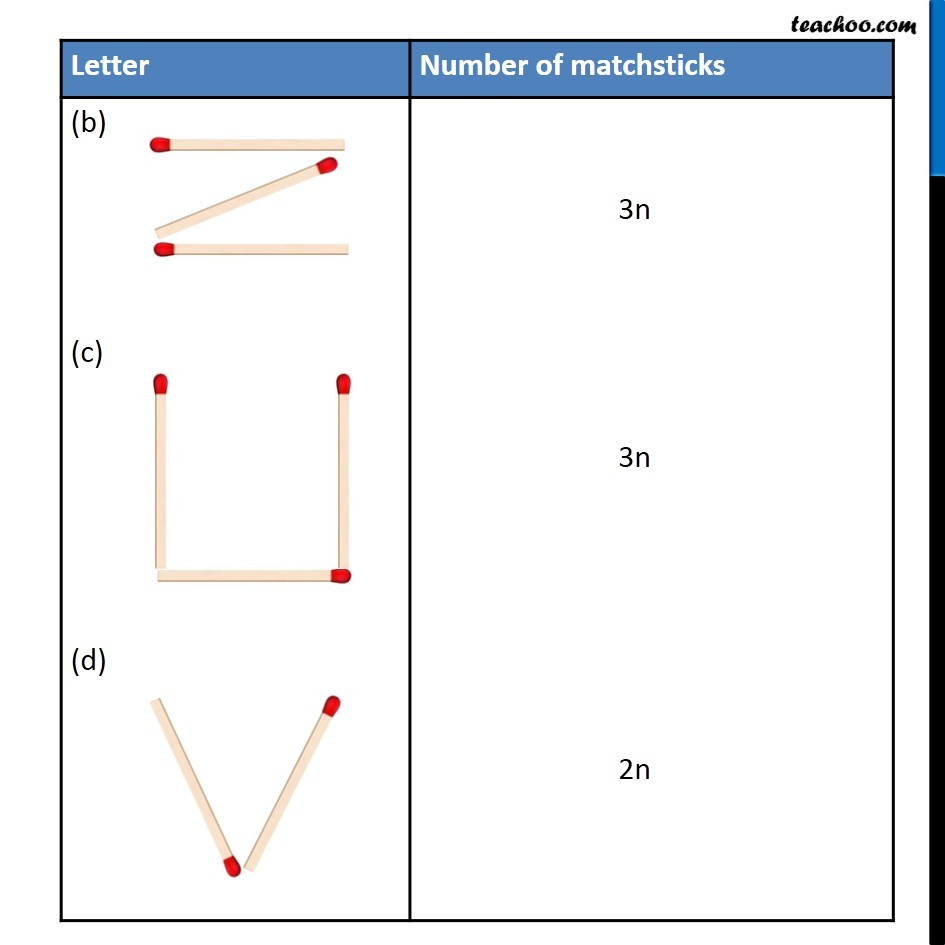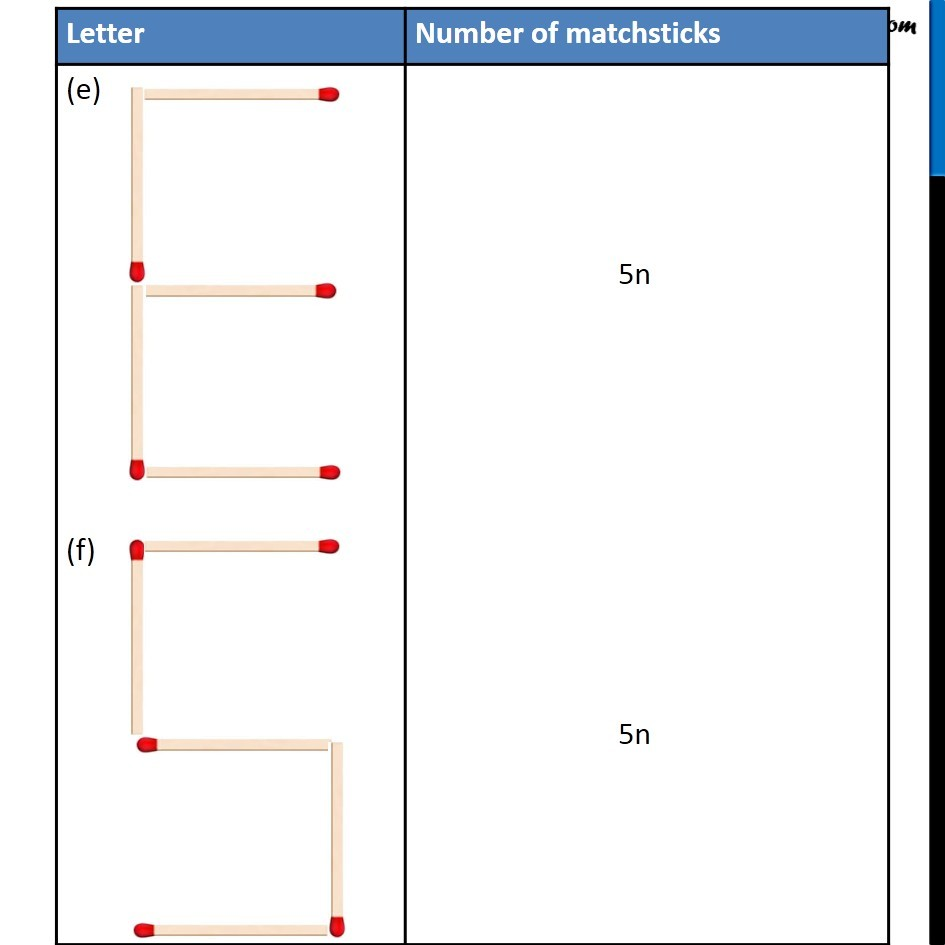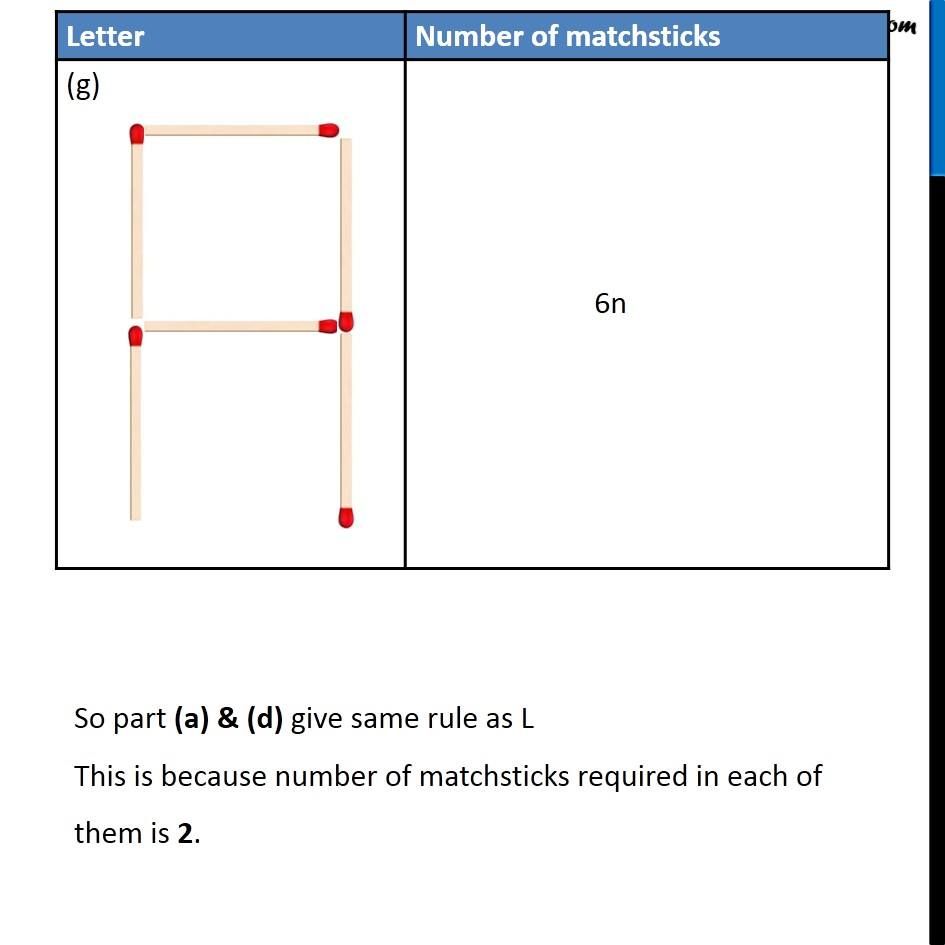1. Chapter 11 Class 6 Algebra
2. Concept wise
3. Matchstick pattern

Transcript

Ex 11.1, 2 We already know the rule for the pattern of letters L, C and F. Some of the letters from Q.1 (given above) give us the same rule as that given by L. Which are these? Why does this happen ? Rule for L is Number of matchsticks = 2n In Part (i) So part (a) & (d) give same rule as L This is because number of matchsticks required in each of them is 2.

Matchstick pattern